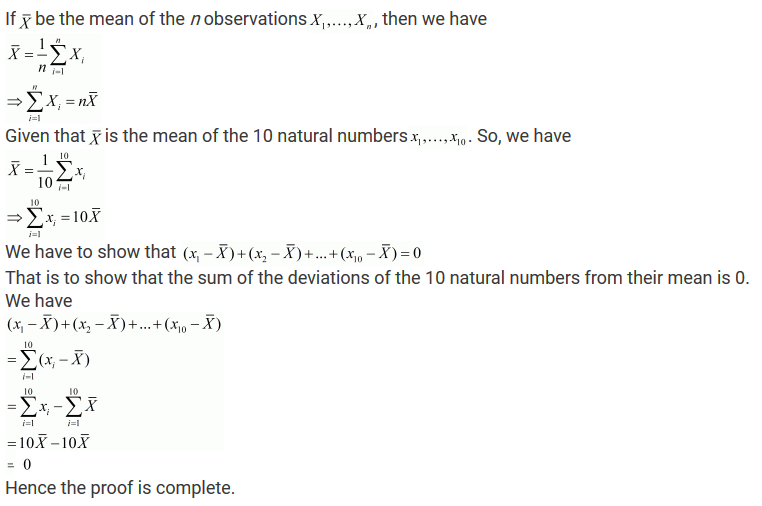#### Chapter 24 Measure of Central Tendency R.D. Sharma Solutions for Class 9th Exercise 24.1

Exercise 24.1

1. If the height of 5 persons are 140 cm, 150 cm, 152 cm, 158 cm and 161 cm respectively, find the mean height.

Solution

Given,
The heights of 5 persons are 140 cm , 150 cm , 152 cm , 158 cm and 161 cm
∴ Mean Weight = sum of heights / total no. of persons
= 140+150+152+158+161/5
= 761/5 = 152.2

2. Find the mean of 994, 996, 998, 1002, 1000.

Solution

Given,
Numbers are 994,996,998,1000,1002
∴ Mean Weight = sum of heights/total no. of persons
= 994+996+998+1000+1002/5
= 4990/5 = 998
Mean = 998

3. Find the mean of first five natural numbers.

Solution

The first five odd numbers are 1 , 2 , 3 , 4 , 5.
∴ Mean = sum of numbers/total numbers
= 1+2+3+4+5/5
= 15/5 = 3
Mean = 3

4. Find the mean of all factors of 10.

Solution

All factors of 6 are 1 , 2 , 5 , 10.
∴ Mean = sum of factors/ total factors
= 1+2+5+10/4 = 4.5
Mean = 4.5

5. Find the mean of first 10 even natural numbers.

Solution

The first five even natural numbers are 2 , 4 , 6 , 8 , 10 , 12 , 14 , 16 , 18 , 20
∴ Mean = sum of numbers / total numbers
= 2+4+6+8+10+12+14+16+18+20/10 = 11
Mean = 11

6.  Find the mean of x, x+2, x+4, x+6, x+8.

Solution

Numbers are x , x + 2 , x + 4 , x + 6 , x + 8.
∴ Mean = sum of numbers total numbers
= x + x + 2 + x + 4 + x + 6 + x + 85
= 5x + 20/5
= 5(x+4/5)
= x + 4

7. Find the mean of first five multiples of 3.

Solution

First five multiples of 3 are 3 , 6 , 9 , 12 , 15.
∴ Mean = sum of numbers/total numbers
= 3+6+9+12+15/5
= 9
Mean = 9

8. Following are the weights of 10 new born babies in a hospital on a particular day : 3.4 , 3 .6 , 4.2 , 4.5 , 3.9 , 4.1 , 3.8 , 4.5 , 4.4 , 3.6 (in kg). Find the mean.

Solution

The weights (in kg) of 10 new born  babies are : 3.4 , 3 .6 , 4.2 , 4.5 , 3.9 , 4.1 , 3.8 , 4.5 , 4.4 , 3.6
Mean Weight = sum of weights/total no.of babies
= 3.4+3.6+4.2+4.5+3.9+4.1+3.8+4.5+4.4+3.610
= 4 kg

9. The percentage marks obtained by students of a class in mathematics are as follows: 64 , 36 , 47 , 23 , 0 , 19 , 81 , 93 , 72 , 35 , 3 , 1 . Find their mean.

Solution

The percentage marks obtained by students are 64 , 36 , 47 , 23 , 0 , 19 , 81 , 93 , 72 , 35 , 3 , 1
∴ Mean marks = sum of marks/total numbers of marks
= 64+36+47+23+0+19+81+93+72+35+3+1/5 =39.5
Mean Marks = 39.5

10. The numbers of children in 10 families of a locality are 2 , 4 , 3 , 4 , 2 , 3 , 5 , 1 , 1 , 5 . Find the mean number of children per family.

Solution

The numbers of children  in 10 families  are : 2 , 4 , 3 , 4 , 2 , 3 , 5 , 1 , 1 , 5
∴ Mean = total no.children/total families
= 2+4+3+4+2+3+5+1+1+5/10 = 3

11. If M is the mean of x1, x2, x3, x, x5 and x6, prove that
(x1 − M) + (x2 − M) (x3 − M) (x4 − M) + (x5 − M) + (x6 − M) = 0
.

Solution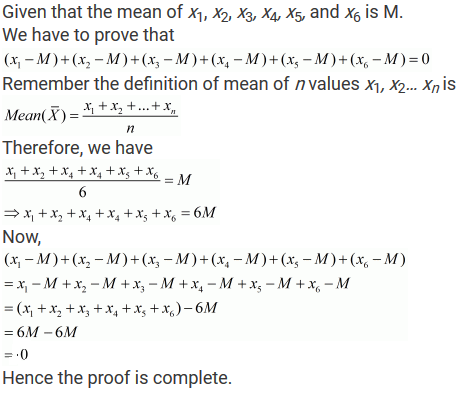12. Durations of sunshine (in hours) in Amritsar to first 10 days of August 1997 as reported by the Meteorological Department are given below:
9.6, 5.2, 3.5, 1.5, 1.6, 2.4, 2.6, 8.4, 10.3, 10.9
(i) Find the mean x̅ .Solution

Given that the duration of sunshine (in hours) in Amritsar for 10 days are 9.6, 5.2, 3.5, 1.5, 1.6, 2.4, 2.6, 8.4, 10.3, and 10.9.

(i) We have to find their mean.
Remember the definition of mean of n values x1, x2… xn is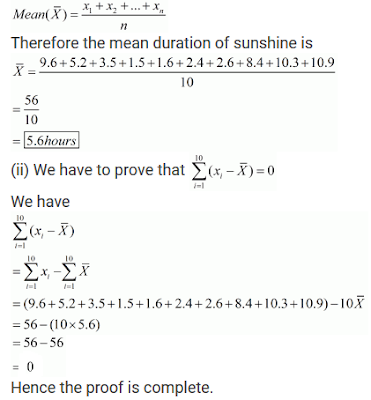13. Explain, by taking a suitable example, how the arithmetic mean alters by (i) adding a constant k to each term, (ii) subtracting a constant k from each them, (iii) multiplying each term by a constant k and (iv) dividing each term by a non-zero constant k.

Solution

Let say numbers are 3 , 4 , 5
∴ Mean = sum of numbers total numbers
= 3+4+5/3 = 4 .

(i) Adding constant term k = 2 in each term.
New numbers are = 5 , 6 , 7
∴ Mean = sum of numbers total numbers
= 5+6+7/3
= 6 = 4 + 2
∴ new  mean will be 2 more than the original mean.

(ii) Subtracting constant term k = 2 in each term.
New numbers are = 1 , 2 , 3
∴ Mean    = sum of numbers total numbers
= 1+2+3/3
= 2 = 4 – 2

∴ new  mean will be 2 less than the original mean

(iii) Multiplying by constant term k = 2 in each term.
New numbers are = 6 , 8 , 10
∴ Mean    = sum of numbers total numbers
= 6+8+10/3
= 8 = 4×2
∴ new  mean will be 2 times of  the original mean.

(iv) Divide the constant term k =2 in each term.
New numbers are = 1.5 , 2 , 2.5.
∴ Mean    = sum of numbers/total numbers
= 1.5+2+2.53
= 2 = 42
∴ new  mean will be half  of  the original mean.

14. The mean of marks scored by 100 students was found to be 40. Later on, it was discovered that a score of 53 was misread as 83. Find the correct mean.

Solution

Mean marks of 100 students = 40
Sum of marks of 100 students = 100×40
= 4000
Correct value = 53
Incorrect value = 83
Correct sum = 4000 – 83 + 53 = 3970
∴ correct mean = 3970/100 = 39.7

15. The traffic police recorded the speed (in km/hr) of 10 motorists as 47 , 53 , 49 , 60 , 39 , 42 , 55 , 57 , 52 , 48 . Later on, an error in recording instrument was found. Find the correct average speed of the motorists if the instrument is recorded 5 km/hr less in each case.

Solution

The speed of 10 motorists are 47 , 53 , 49 , 60 , 39 , 42 , 55 , 57 , 52 , 48 .
Later on it was discovered that the instrument recorded 5 km/hr less than in each case
∴ correct values are = 52 , 58 , 54 , 65 , 44 , 47 , 60 , 62 , 57 , 53.
∴ correct mean = 52+58+54+65+44+47+60+62+57+53/10
= 552/10 =55.2 km/hr .

16. The mean of five numbers is 27. If one number is excluded, their mean is 25. Find the excluded number.

Solution

The mean of five numbers is 27
The sum of five numbers = 5×27 = 135
If one number is excluded , the new mean is 25
∴ Sum of 4 numbers = 4×25 = 100
∴ Excluded number = 135 – 100 = 35

17. The mean weight per student in a group of 7 students is 55 kg. The individual weights of 6 of them (in kg) are 52, 54, 55, 53, 56 and 54. Find the weight of the seventh student.

Solution

The mean weight per student in a group of 7 students = 55 kg
Weight of 6 students (in kg) = 52 , 54 , 55 , 53 , 56 and 54
Let the weight of seventh student = x kg
∴ Mean Weight = sum of weights/total no.of students
⇒ 55 = (52+54+55+53+56+54+x)/ 7
⇒ 385 = 324 + x ⇒ x = 385−324
⇒ x = 61 kg
∴ weight of seventh student = 61 kg.

18. The mean weight of 8 numbers is 15. If each number is multiplied by 2 , what will be the new mean ?

Solution

We have ,
The mean weight of 8 numbers is 15
Then , the sum of 8 numbers = 8×15 = 120
If each number is multiplied by 2
Then , new mean = 120×2 = 240
∴ New  mean = 240/8 = 30.

19. The mean of 5 numbers is 18. If one number is excluded, their mean is 16. Find the excluded number.

Solution

The mean of 5 numbers is 18
Then , the sum of 5 numbers = 5×18 = 90
If one number is excluded
Then , the mean of 4 numbers = 16
∴ sum of 4 numbers = 4×16 = 64
Excluded number = 90 – 64 = 26.

20. The mean of 200 items was 50. Later on, it was on discovered that the two items were misread as 92 and 8 instead of 192 and 88. Find the correct mean.

Solution

The mean of 200 items = 50
Then the sum of 200 items = 200×50 = 10,000
Correct values = 192 and 88.
Incorrect values = 92 and 8.
∴ correct sum = 10000 - 92 - 8 + 192 + 88 = 10180
∴ correct mean = 10180/200 = 101.8/2 = 50.9 .

21. Find the values of n and X in each of the following cases: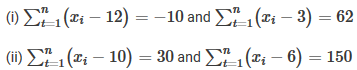Solution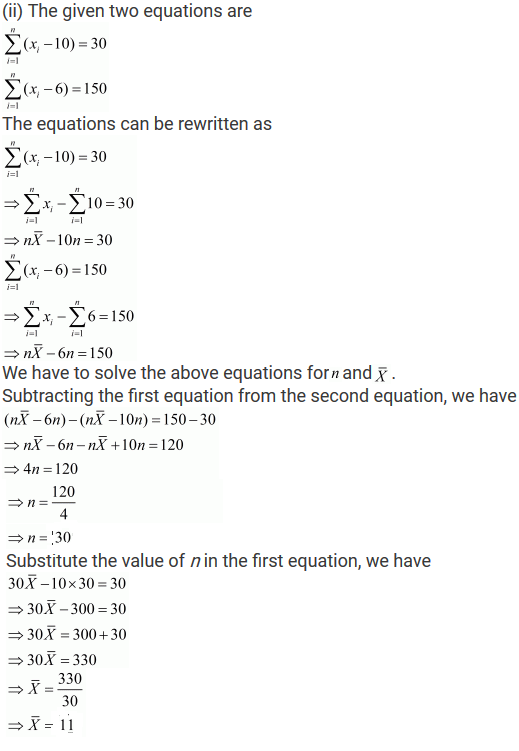22. The sum of the deviations of a set of n values x1, x2,...., xn, measured from 15 and -3 are -90 and 54 respectively. Find the value of n and mean.

Solution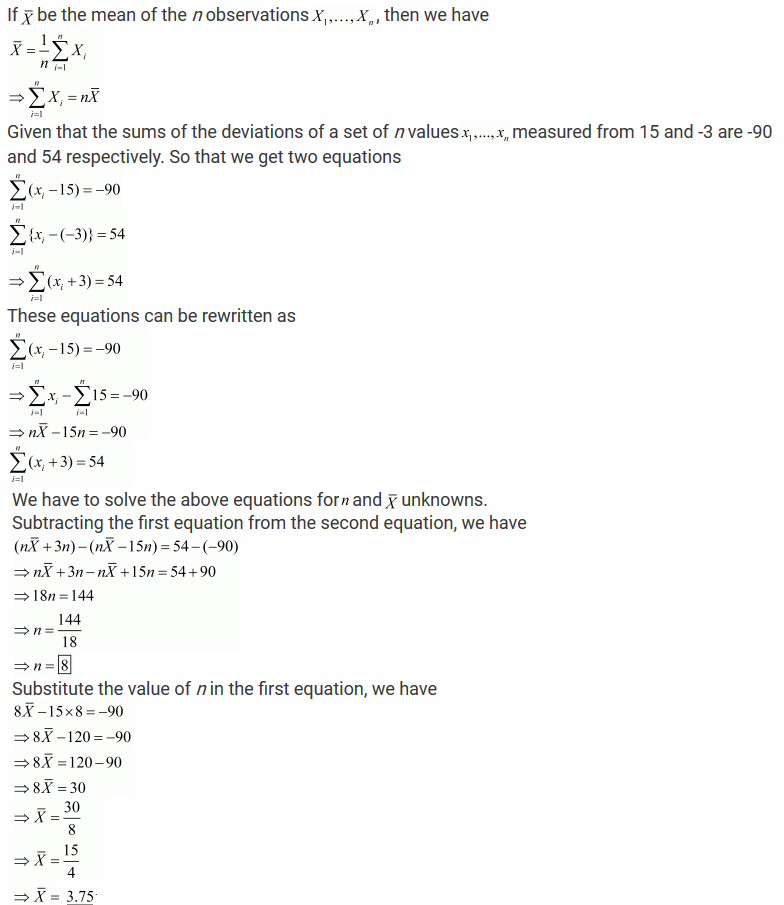23. Find the sum of the deviations of the variate values 3, 4, 6, 7, 8, 14 from their mean.

Solution24. If x̅ is the mean of the ten natural numbers x1, x1, x1 ....,x1, Show that (x1 - x̅) + (x2 - x̅) + ... + (x10 - x̅) = 0.

Solution